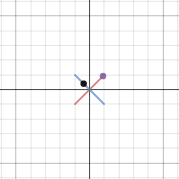Dear Uncle Colin,

I was playing with parametric equations and stumbled on something Wolfram Alpha wouldn’t plot: $x=t^i;\, y = t^{-i}$.

Does this curve really exist? Or am I imagining it?

-- A Real Graph? A Non-existant Drawing?

Hi, ARGAND – what you’re trying to plot certainly exists; whether or not it’s a (real) curve is doubtful.

Before we get to trying to draw the graph, we need to figure out what $t^i$ means – can we reasonably take the $i$th power of something?

Well, thanks to Euler, it turns out we can. Our buddy Len said $e^{i\theta} \equiv \cos(\theta) + i \sin(\theta)$, so if we let $t = e^{\theta}$, we get $x = \cos(\theta) + i\sin(\theta)$ and $y =\cos(\theta) - i\sin(\theta)$, where $\theta = \ln(t)$.

If we’re looking for real points, $\sin(\theta)$ – that is to say, $\sin(\ln(t))$ – has to vanish, which it does when $\ln(t) = n\pi, \, n \in \bb{N}$.

When $n$ is even, $\cos(\ln(t)) = 1$; otherwise, $\cos(\ln(t)) = -1$, so we have something that’s technically a function, even though it exists at only two points: $(-1,-1)$ and $(1,1)$.

It would take a great deal of imagination to call that a curve!

If we branch out into the complex plane, $z = t^i$ and $z=t^{-i}$ both trace out the unit circle, in different directions. When $t = \frac {\pi}{4}$, for example, $x = \frac{1}{\sqrt{2}} + \frac{1}{\sqrt{2}} i$ and $y = \frac{1}{\sqrt{2}} - \frac{1}{\sqrt{2}}i$.

However, trying to plot the complex curve would require four dimensions, which is more than I can comfortably hold in my head. It’s easy enough to plot the real part, though: that’s a straight line between (1,1) and (-1,-1). The imaginary part is also a straight line, between (-1,1) and (1,-1). It’s fitting the two together in four-space that’s the trouble!-- Uncle Colin